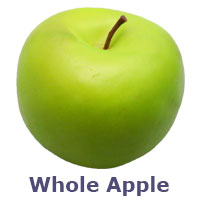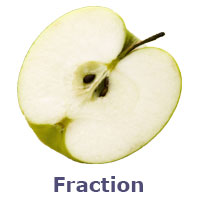Whole numbersA number, which represents either whole or none is defined, a whole number.

The meaning of whole is complete. So, it should be used in dealing whole quantities and should not be used in the case of fractions. Whole numbers are basically natural numbers but a number called zero $\left(0\right)$ is joined in that group to form new numbers group. Therefore, the collection of natural numbers along with zero is called whole numbers.

The purpose of these numbers is same as the natural numbers but the specialty of this group is zero. In other words, whole numbers are used to count whole quantities, measuring whole quantities and labelling whole quantities. If none is there, then it is represented by the zero mathematically.

The system of whole numbers was developed by using numerals of Indo-Arabic Numeral system. The starting number of the whole numbers is zero and numeral $1$ has to add to each number to get the next whole number. It is continued infinitely. Thus the whole numbers group was introduced in number system.

How to use

Whole numbers are actually used to represent whole quantities. There is an apple which is whole. So, the number can be represented by the whole numbers system. Hence, it is known as $1$ apple. There is also apple but it is not whole, means fraction. So, it cannot be said as $1$ apple. Therefore, use whole numbers always to represent whole quantities.There are two footballs in second example. So, whole numbers is used to represent the number of things. Hence, it is called as two footballs. Similarly, there is no football. The whole number $0$ is used to represent nothing or none. Hence, it is called as $0$ football.These two examples cleared that whole numbers are always used to represent whole quantities and never represent fractions. Similarly, whole numbers are not only used to represent whole things and also used to represent none or nothing mathematically with zero.

Representation

The collection of whole numbers is a set. The set of whole numbers is denoted by the letter $W$ and it is written in set form in mathematics.

$W=\left\{0,1,2,3,\dots \right\}$

Whole numbers starts from zero and the collection is continued infinitely. So, the set of whole numbers is known as an infinite set.

Latest Math Topics
Latest Math Problems
Email subscription
Math Doubts is a best place to learn mathematics and from basics to advanced scientific level for students, teachers and researchers. Know more